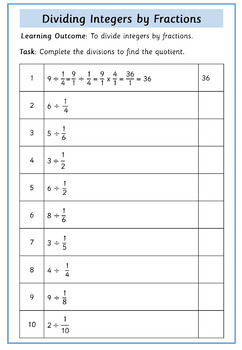# Dividing FractionsSubject
Resource Type
File Type

Zip

(1 MB|15 pages)
Standards
Also included in:
1. This resource contains a 54 slide PowerPoint lesson and 15 worksheets on dividing fractions.The PowerPoint visually explains and demonstrates step-by-step how to divide fractions. Students are given opportunities to carry out the division of fractions and to spot errors in calculations.The bundle al
\$7.49
\$3.99
Save \$3.50
• Product Description
• StandardsNEW

This resource contains a 15-page workbook / 15 worksheets (with answers) on dividing fractions. Tasks include:

• dividing whole numbers by fractions
• dividing fractions by whole numbers
• dividing fractions by fractions
• dividing fractions by mixed numbers
• dividing mixed numbers by fractions
• dividing mixed numbers by mixed numbers
• solving word problems involving the division of fractions.

You may also be interested in:

Dividing Fractions - 54 Slide PowerPoint Lesson

This resource contains a 54 slide PowerPoint lesson on dividing fractions. It visually explains and demonstrates step-by-step how to divide fractions. The presentation includes:

dividing whole numbers by fractions
dividing fractions by whole numbers
dividing fractions by fractions
dividing fractions by mixed numbers
dividing mixed numbers by fractions
dividing mixed numbers by mixed numbers

Students are given opportunities to carry out the division of fractions and to spot errors in calculations.

Save money and buy both resources together at a discounted rate:

Dividing Fractions - 54 Slide PowerPoint Lesson and 15 Worksheets

More Fraction Resources

Follow me here to be kept informed of free and new resources.

Interpret and compute quotients of fractions, and solve word problems involving division of fractions by fractions, e.g., by using visual fraction models and equations to represent the problem. For example, create a story context for (2/3) ÷ (3/4) and use a visual fraction model to show the quotient; use the relationship between multiplication and division to explain that (2/3) ÷ (3/4) = 8/9 because 3/4 of 8/9 is 2/3. (In general, (𝘢/𝘣) ÷ (𝘤/𝘥) = 𝘢𝘥/𝘣𝘤.) How much chocolate will each person get if 3 people share 1/2 lb of chocolate equally? How many 3/4-cup servings are in 2/3 of a cup of yogurt? How wide is a rectangular strip of land with length 3/4 mi and area 1/2 square mi?
Solve real world problems involving division of unit fractions by non-zero whole numbers and division of whole numbers by unit fractions, e.g., by using visual fraction models and equations to represent the problem. For example, how much chocolate will each person get if 3 people share 1/2 lb of chocolate equally? How many 1/3-cup servings are in 2 cups of raisins?
Interpret division of a whole number by a unit fraction, and compute such quotients. For example, create a story context for 4 ÷ (1/5), and use a visual fraction model to show the quotient. Use the relationship between multiplication and division to explain that 4 ÷ (1/5) = 20 because 20 × (1/5) = 4.
Interpret division of a unit fraction by a non-zero whole number, and compute such quotients. For example, create a story context for (1/3) ÷ 4, and use a visual fraction model to show the quotient. Use the relationship between multiplication and division to explain that (1/3) ÷ 4 = 1/12 because (1/12) × 4 = 1/3.
Apply and extend previous understandings of division to divide unit fractions by whole numbers and whole numbers by unit fractions.
Total Pages
15 pages
Included
Teaching Duration
N/A
Report this Resource to TpT
Reported resources will be reviewed by our team. Report this resource to let us know if this resource violates TpT’s content guidelines.\$2.80
List Price:
\$3.50
You Save:
\$0.70